A few months ago I wrote a post on formulating the Normal Equation for linear regression. A crucial part in the formulation is using matrix calculus to compute a scalar-by-vector derivative. I didn't spend much time explaining how this step works, instead remarking:

Deriving by a vector may feel uncomfortable, but there's nothing to worry about. Recall that here we only use matrix notation to conveniently represent a system of linear formulae. So we derive by each component of the vector, and then combine the resulting derivatives into a vector again.

According to the comments received on the post, folks didn't find this convincing and asked for more details. One commenter even said that "matrix calculus feels handwavy", something which I fully agree with. The reason matrix calculus feels handwavy is that it's not as commonly encountered as "regular" calculus, and hence its identities and intuitions are not as familiar. However, there's really not that much to it, as I want to show here.

Let's get started with a simple example, which I'll use to demonstrate the principles. Say we have the function: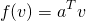Where a and v are vectors with n components . We want to compute its derivative by v. But wait, while a "regular" derivative by a scalar is clearly defined (using limits), what does deriving by a vector mean? It simply means that we derive by each component of the vector separately, and then combine the results into a new vector . In other words: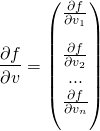Let's see how this works out for our function f. It may be more convenient to rewrite it by using components rather than vector notation: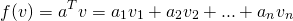Computing the derivatives by each component, we'll get: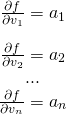So we have a sequence of partial derivatives, which we combine into a vector: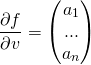Or, in other words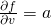.

This example demonstrates the algorithm for computing scalar-by-vector derivatives:

1. Figure out what the dimensions of all vectors and matrices are.
2. Expand the vector equations into their full form (a multiplication of two vectors is either a scalar or a matrix, depending on their orientation, etc.) Note that this will end up with a scalar.
3. Compute the derivative of the scalar by each component of the variable vector separately.
4. Combine the derivatives into a vector.

Similarly to regular calculus, matrix and vector calculus rely on a set of identities to make computations more manageable. We can either go the hard way (computing the derivative of each function from basic principles using limits), or the easy way - applying the plethora of convenient identities that were developed to make this task simpler. The identity for computing the derivative of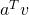shown above plays the role of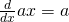in regular calculus.

Now we have the tools to understand how the vector derivatives in the normal equation article were computed. As a reminder, this is the matrix form of the cost function J: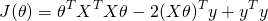And we're interested in computing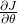. The equation for J consists of three terms added together. The last one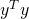doesn't contribute to the derivative because it doesn't depend on the variable. Let's start looking at the second (since it's simpler than the first) - and give it a name, for convenience: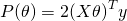We'll start by recalling what all the dimensions are.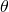is a vector of n components.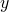is a vector of m components.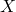is a m-by-n matrix.

Let's see what P expands to :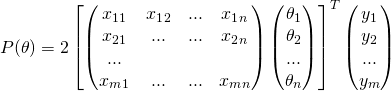Computing the matrix-by-vector multiplication inside the parens: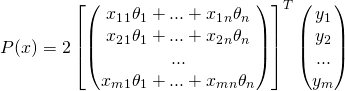And finally, multiplying the two vectors together: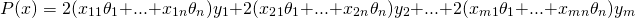Working with such formulae makes you appreciate why mathematicians have long ago come up with shorthand notations like "sigma" summation: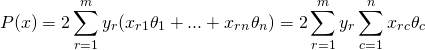OK, so we've finally completed step 2 of the algorithm - we have the scalar equation for P. Now it's time to compute its derivative by each: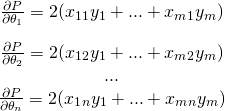Now comes the most interesting part. If we treat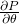as a vector of n components, we can rewrite this set of equations using a matrix-by-vector multiplication: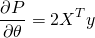Take a moment to convince yourself this is true. It's just collecting the individual components of X into a matrix and the individual components of y into a vector. Since X is a m-by-n matrix and y is a m-by-1 column vector, the dimensions work out and the result is a n-by-1 column vector.

So we've just computed the second term of the vector derivative of J. In the process, we've discovered a useful vector derivative identity for a matrix X and vectorsand y: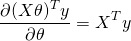OK, now let's get back to the full definition of J and see how to compute the derivative of its first term. We'll give it the name Q: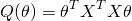This derivation is somewhat more complex, sinceappears twice in the equation. Here's the equation again with all the matrices and vectors fully laid out (note that I've already done the transposes):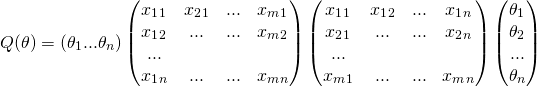I'll just multiply the two matrices in the middle together. The result is a "X squared" matrix, which is n-by-n. The element in row r and column c of this square matrix is: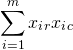Note that "X squared" is a symmetric matrix (this fact will be important later on). For simplicity of notation, we'll call its elements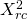. Multiplying by thevector on the right we get: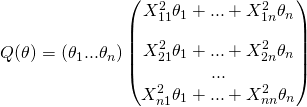And left-multiplying byto get the fully unwrapped formula for Q: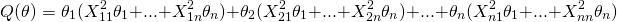Once again, it's now time to compute the derivatives. Let's focus on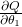, from which we can infer the rest: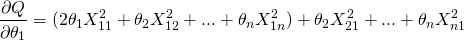Using the fact that X squared is symmetric, we know that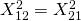and so on. Therefore: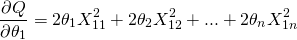The partial derivatives by othercomponents are similar. Collecting the sequence of partial derivatives back into a vector equation, we get: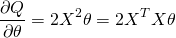Now back to J. Recall that for convenience we broke J up into three parts: P, Q and; the latter doesn't depend onso it doesn't play a role in the derivative. Collecting our results from this post, we then get: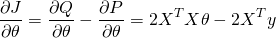Which is exactly the equation we were expecting to see.

To conclude - if matrix calculus feels handwavy, it's because its identities are less familiar. In a sense, it's handwavy in the same way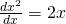is handwavy. We remember the identity so we don't have to recalculate it every time from first principles. Once you get some experience with matrix calculus, parts of equations start looking familiar and you no longer need to engage in the long and tiresome computations demonstrated here. It's perfectly fine to just remember that the derivative of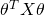with a symmetric X is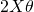. See the "identities" section of the wikipedia article on matrix calculus for many more examples.

  A few words on notation: by default, a vector v is a column vector. To get its row version, we transpose it. Moreover, in the vector derivative equations that follow I'm using denominator layout notation. This is not super-important though; as the Wikipedia article suggests, many mathematical papers and writings aren't consistent about this and it's perfectly possible to understand the derivations regardless.
  Yes, this is exactly like computing a gradient of a multivariate function.
  Take a minute to convince yourself that the dimensions of this equation work out and the result is a scalar.